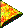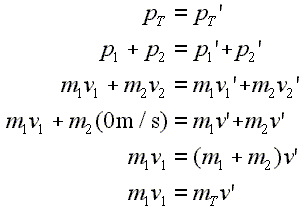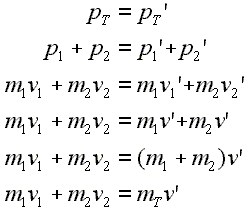# Introductory Momentum Equations, Two Body, Stick TogetherStick Together Problem

Suppose that one object is moving along at constant velocity, and it strikes a stationary object such that the two objects stick together after the collision and, effectively, move as a single object afterwards. One could imagine a train car rolling along and running into another car that is standing still. Their couplers would connect, and they would roll away hooked together as one object. As in any collision, momentum would be conserved.

Here is a animation demonstrating this problem:

These are the equations for this situation:Lines 1, 2, and 3: Basic start for two body problem. Line 4: Original velocity for second object is set to zero. Both final velocities are set to the same value. Line 5: Term for original momentum of second object drops out. Common final velocity terms are collected. Line 6: The sum of the masses is expressed as mass total.

As shown on the page before this, the first three lines begin the analysis of most two body momentum problems.

Since the second object is standing still before the collision, its velocity is set to zero causing its term to drop out of the equation. This happens in line four. Also in line three both of the final velocities are set to the same value, v'. They are both set to the same value because the two objects stick together, moving with the same mutual velocity. We will call this mutual velocity v'.

In line five the term for the original velocity of the second object has dropped out; it was equal to zero since the velocity of this object is zero before the collision. Also, in this line the terms on the right side are collected showing that the sum of the masses is multiplied by the mutual velocity to equal the total momentum of the system after the collision.

In line six, the last line, the addition of the two masses is replaced with the variable MT which stands for the total mass of the two objects after they have stuck together. In this condition the two objects move along as if they were one object.

The problem is not much harder if the second object is not standing still before the collision, but is in motion. Of course, momentum is still conserved. The physics equations for these conditions would look like the following.Lines 1, 2, and 3: Basic start for two body problem. Line 4: Both final velocities are set to the same value. Line 5: Mutual final velocity is factored out of each term on the right. Line 6: The sum of the masses is expressed as mass total.

An animation for such a situation would look like this:

Related Problems

     

Do not confuse either of these stick together type problems with situations where objects collide and then bounce off of one another. Momentum is conserved in both types, but the problems where the objects bounce apart after the collision can get more complicated. We will take a look at such conditions later.

Custom Search